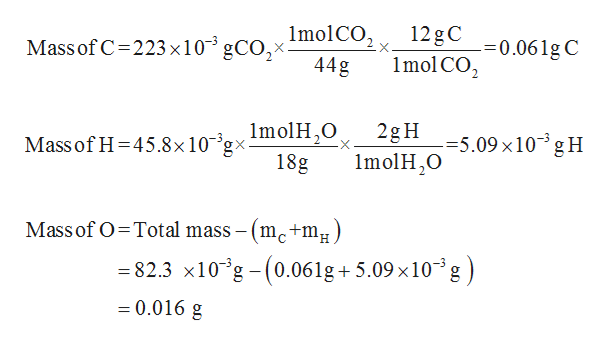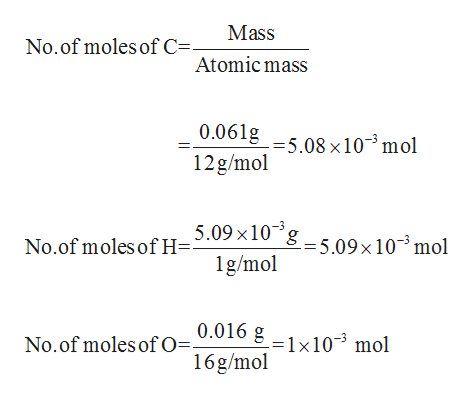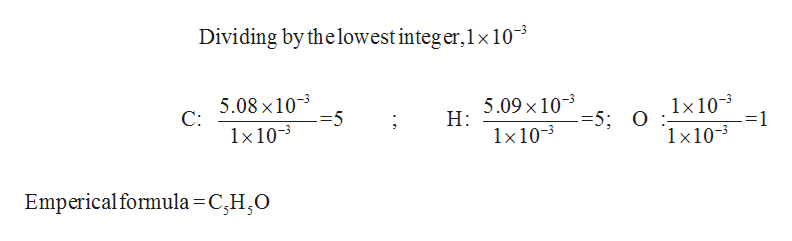# A compound containing only C, H, and O, was extracted from the bark of the sassafras tree. The combustion of 82.3mg produced 223mg of CO2and 45.8mg of H2O. The molar mass of the compound was 162 g/mol. Determine its empirical and molecular formulas.(For this problem use the format: CxHyOz, where x, y, and z are subscripts of 2 or greater.)Thank you

Question
16 views

A compound containing only C, H, and O, was extracted from the bark of the sassafras tree. The combustion of 82.3mg produced 223mg of CO2and 45.8mg of H2O. The molar mass of the compound was 162 g/mol. Determine its empirical and molecular formulas.

(For this problem use the format: CxHyOz, where x, y, and z are subscripts of 2 or greater.)

Thank you

check_circle

star
star
star
star
star
1 Rating
Step 1

Given that the mass of CO2 and H2O formed by the burning of 82.3 mg of compound is 223 mg and 45.8 mg respectively.

The mass of Carbon, Hydrogen and oxygen can be calculated using the simple mole concept.help_outlineImage TranscriptioncloseImolCO,, 12gC Massof C=223 ×10° gCO,× -=0.061g C 1mol CO, 44g Massof H=45.8×10-°9xImolH,0 18g 2gH =5.09×10³gH ImolH,0 Mass of O=Total mass – (mc+mµ. = 82.3 x10°g - (0.061g+ 5.09 x10°g ) %3D = 0.016 g %3D fullscreen
Step 2

Number of moles of Carbon, Hydrogen and oxygen can be calculated by the equation given below:help_outlineImage TranscriptioncloseMass No.of moles of C=- Atomic mass 0.061g -=5.08 x10- mol 12g/mol 5.09 x10 ´g – 5.09×10³mol H= 1g/mol No.of moles of 0.016 g No.of moles of O=. =1x10-³ mol 16g/mol fullscreen
Step 3

In-order to get the empirical formula, first the number of moles has to be divided with the lowest...help_outlineImage TranscriptioncloseDividing by thelowest integer,1x 10-3 5.08 x103 C: 5.09 x103 Н: 1x103 =1 1×10-3 =5 =5; 0 :- 1x10-3 1x10-3 Emperical formula=C,H,O fullscreen

### Want to see the full answer?

See Solution

#### Want to see this answer and more?

Solutions are written by subject experts who are available 24/7. Questions are typically answered within 1 hour.*

See Solution
*Response times may vary by subject and question.
Tagged in

### General Chemistry